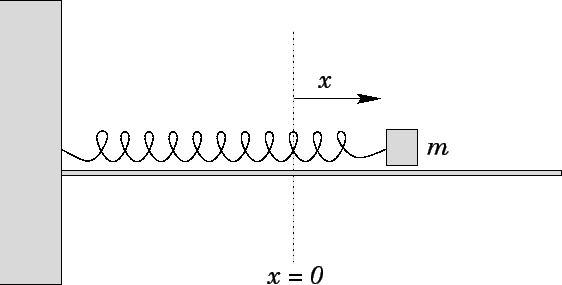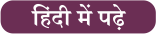Latest SSC jobs   »   Hooke’s Law: Definition And Formula

# Hooke’s Law: Definition And Formula

## Hooke’s Law

The elastic behaviour of a solid is determined by the law of elasticity represented by Hooke’s law. The law is used to find the relation between the force and distance of spring in various branches of science and engineering. This article will highlight the Hooke’s Law Definition, Statement, Formula and applications.

### Hooke’s Law Definition

Hooke’s law discovered by the English scientist Robert Hooke states that the force needed to extend or compress a spring by some distance is proportional to that distance. Hooke’s law holds importance where an elastic body is deformed and by using it you can deduce the relation between strain and stress of complex objects.### Hooke’s Law Formula

Hooke’s law for spring is often stated under the convention that the restoring force exerted by the spring on whatever is pulling its free end. Hence, the formula for Hooke’s law is derived as:where k is the constant factor and x is the distance. The negative sign is used as the direction of the restoring force is opposite to that of the displacement.

RRB NTPC Syllabus: Important Topics

### Hooke’s Law Applications

The applications of Hooke’s law can be seen in our day to day life in many common objects lying in front of you. Some of the applications include:

• Hooke’s law is used to govern the behaviour of springs.
• It is also applied in many other situations where an elastic object is deformed.
• Examples include inflating a balloon, pulling on a rubber band and amount of wind force needed to make a tall building bend etc.

Click here for SSC CGL Tier 2 Free Quizzes of all Topics

### Hooke’s Law FAQs

What does Hooke’s law state?

Hooke’s law simply states the relation between the restoring Force and spring displacement by an equation.

What does F =- KX stand for?

The F =- KX stands for the Hooke’s law that is used to determine the elasticity of an object.## FAQs

### What does Hooke's law state?

Hooke's law simply states the relation between the restoring Force and spring displacement by an equation.

### What does F =- KX stand for?

The F =- KX stands for the Hooke's law that is used to determine the elasticity of an object.

#### Congratulations!General Awareness & Science Capsule PDF